# MOD Function

Returns the remainder after a number (dividend) is divided by another number (divisor)

## What is the MOD Function?

The MOD function is categorized under Excel Math and Trigonometry functions. The function helps find a remainder after a number (dividend) is divided by another number (divisor).

As a financial analyst, the function is useful when we need to process every nth value. For example, if our data comprises several columns of numbers, we can use MOD to sum every nth column.

### Formula

=MOD(number,divisor)

This function uses the following arguments:

1. Number (required argument) – This is the number for which we wish to find the remainder.
2. Divisor (required argument) – This is the number by which we want to divide the number.

### How to use the MOD Function in Excel?

To understand the uses of the MOD function, let’s consider a few examples:

#### Example 1

Suppose we are given the following data: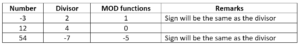In the above data, we used the following formula: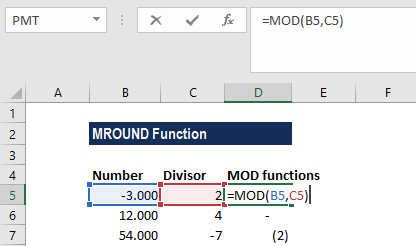The results we get in Excel are shown below: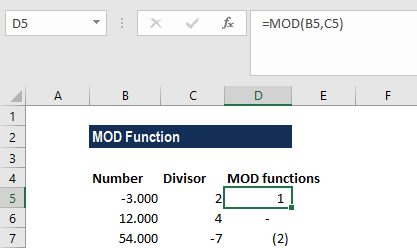#### Example 2

Suppose we are given the following data:If we wish to get the maximum value of every nth column, we can use an array formula that is based on the MAX, MOD, and COLUMN functions.

The formula to use is: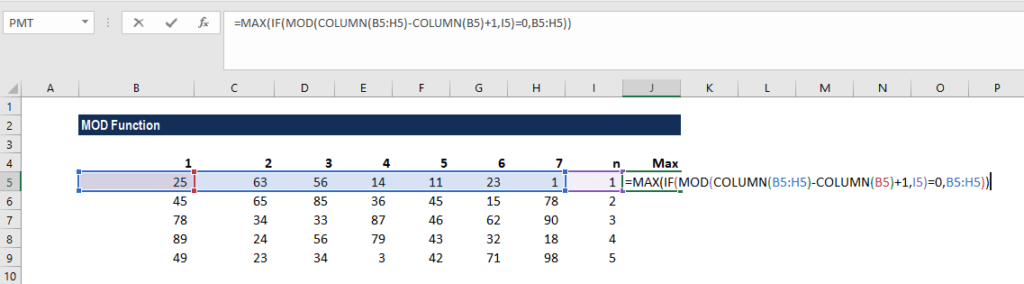As it is an array formula, we need to enter it using Ctrl + Shift + Enter. In the formula above, we used the MOD and COLUMN functions to filter out values not in the nth columns, then run MAX on the result.

We get the result below: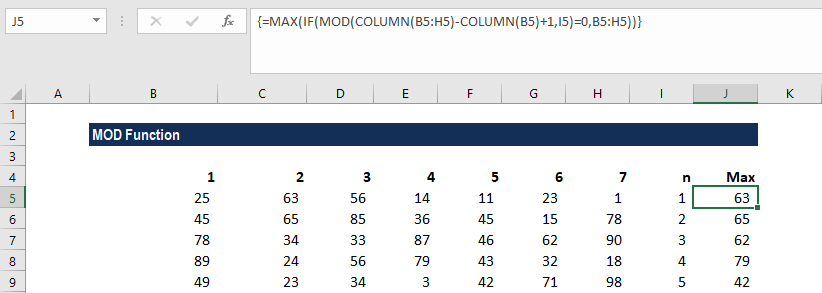Suppose we need to find the MIN function, the formula will be {=MIN(IF(MOD(COLUMN(B5:H5)-COLUMN(B5)+1,I5)=0,B5:H5))}.

Further, if we wish to get the SUMPRODUCT of the even rows, we can use the following formula: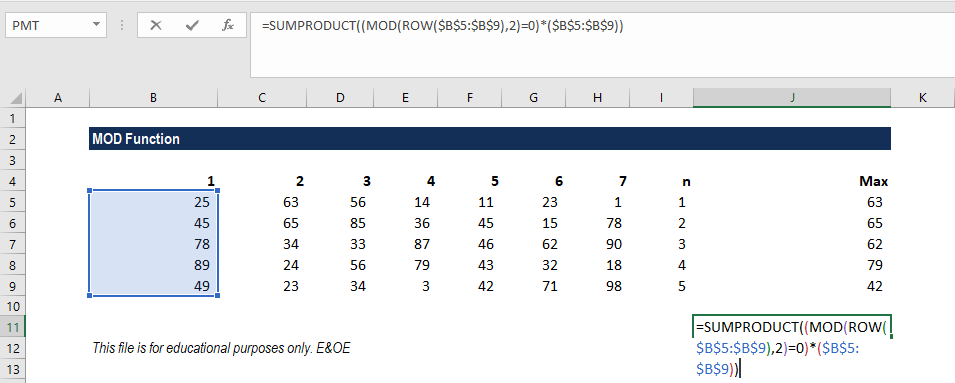We get the result below: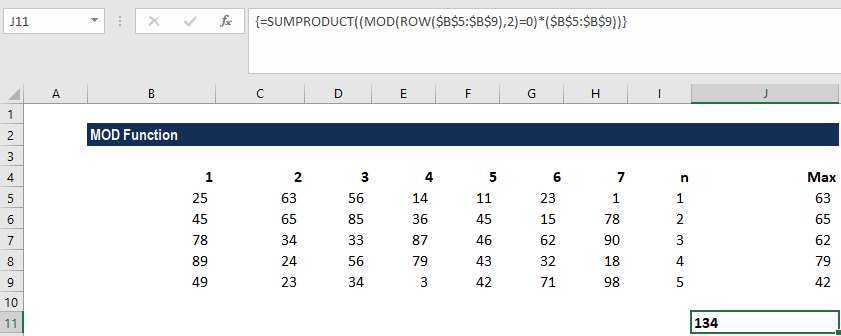For odd rows, the formula will be: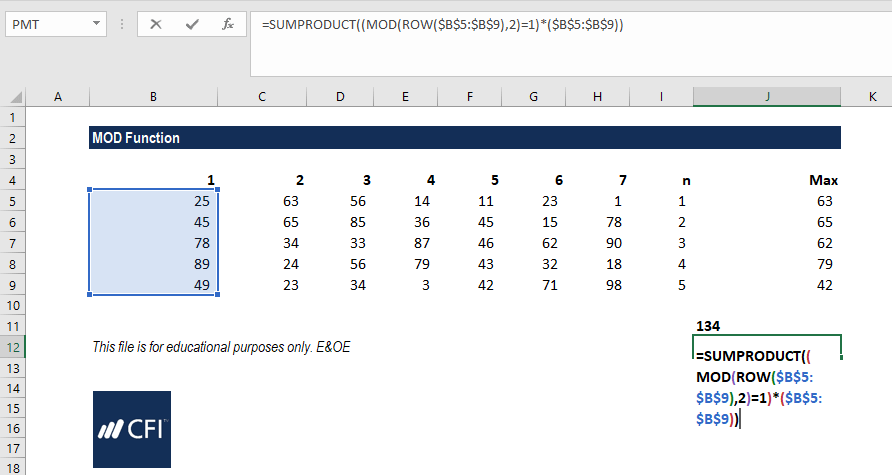We get the result below: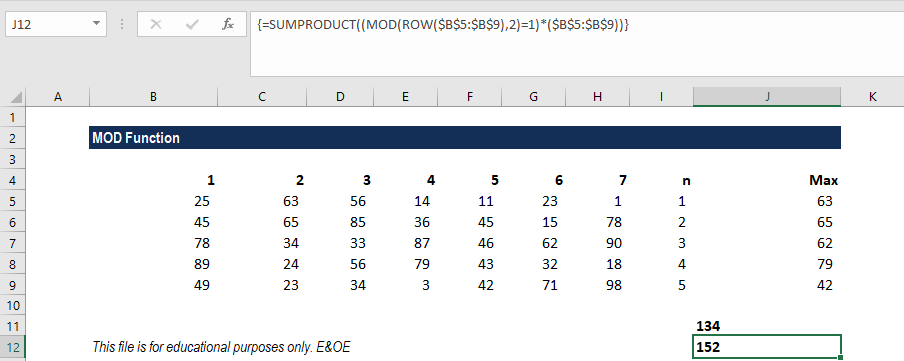### A few things to remember

1. #DIV/0! error – Occurs when the divisor value is zero.
2. The MOD function will return a result in the same sign as the divisor.

Thanks for reading CFI’s guide to important Excel functions! By taking the time to learn and master these Excel functions, you’ll significantly speed up your financial analysis. To learn more, check out these additional CFI resources:

• Excel Functions for Finance